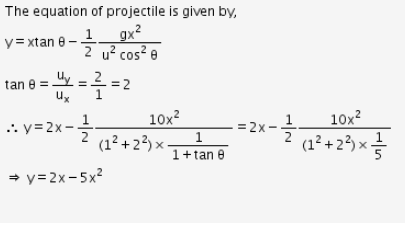# A projectile is given an initial velocity of (i+2j)m/s where i is along the ground and j

A projectile is given an initial velocity of (i+2j)m/s where i is along the ground and j is along the vertical. If g=10m/s2, the equation of its trajectory is?